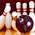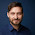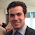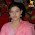## Wednesday, December 26, 2012

### Kernel Approximations for Efficient SVMs (and other feature extraction methods) [update]

Recently we added another method for kernel approximation, the Nyström method, to scikit-learn, which will be featured in the upcoming 0.13 release.
Kernel-approximations were my first somewhat bigger contribution to scikit-learn and I have been thinking about them for a while.
To dive into kernel approximations, first recall the kernel-trick.

### The Kernel Trick

The motivation is to obtain a non-linear decision boundary, because not all problems are linear. Consider this simple 1d dataset

There is obviously no way this can be linearly separated.
There is an easy way to make it linearly separable, though. By embedding the data it in a higher-dimensional space using some non-linear mapping, for example $x \mapsto x^2$

This is obviously a contrived example, but not totally detached from reality.

Learning classifiers in high dimensions is expensive, though, and you have to come up with the non-linear mapping. If you start out with a given number of features $n$ and you want to compute all polynomial terms up to degree 2, you get more than $n^2$ features, and this rises exponentially in the degree $d$ of polynomials you want!

There is a very simple trick to circumvent this problem, though, which is the kernel-trick.
The essence of the kernel-trick is that if you can describe an algorithm in a certain way -- which is using only inner products -- then you never need to actually use the feature mapping, as long as you can compute the inner product in the feature space.

For the polynomial feature map, the inner product in the feature space is given by $k(x, y) = (x^Ty + c)^d$ which is easy enough to compute for any degree $d$.

What is even better is that you don't really need to start from a feature map. You can specify an inner product $k(x, y)$ directly and under mild condition (if $k$ is a Mercer-kernel), there exists a space $H$ for which $k$ is the scalar product. It is possible to construct a mapping from the original $R^n \rightarrow H$ but you never actually need to compute it.

One of the most popular $k$ is the Gaussian (or RBF) kernel
$k(x, y) = \text{exp}(\gamma ||x - y|| ^ 2)$,
which is a scalar product in a space that is even infinite dimensional.

### Kernelized SVMs

One of the most popular applications of the kernel trick is the kernelized Support Vector Machine (SVM), which is one of the best of-the-shelf classifiers today.

One of the characteristics of kernelized algorithms is that their runtime and space complexity is basically independent of the dimensionality of the input space, but rather scales with the number of data points used for training. There are lots of tricks to make SVM training fast, but in general you can assume that the run time is cubic in the number of samples.
Usually good implementations avoid computing the kernel values for all pairs of training points but this comes at the cost of some runtime and algorithmic complexity. If you could afford it, you'd really like to store the whole kernel matrix, i.e. the kernel value for all pairs of training points, which is quadratic in the number of samples.

If you have very complex, but small (say <10.000 samples or <100.000 depending on your patience) kernels are pretty neat. But if you deal with really A LOT of data, there is no way you can train an SVM - it will be just to slow or the memory complexity will just be to high. One trend in recent years has been to apply stochastic gradient descent (SGD) optimization to learn linear classifiers.

These can be really fast - a single iteration is linear in the dimensionality (or rather in the number of non-zero features per sample, which is very nice for sparse data with very few non-zero features per sample) and convergence is sublinear in the number of samples (in theory)!

So that is really, really fast. But classifiers learned in this way are usually linear in the data (except neural networks which I will not talk about much today).

### Kernel approximation - Marrying kernels and SGD

So on the one hand, we have kernelized SVMs, which work quite well on complicated that that is not linearly separable, but doesn't scale well to many samples. On the other hand, we have SGD optimization that is very efficient, but only produces linear classifiers.

A somewhat recent trend is to combine the two by (sort of) moving away from the kernel trick and computing explicit feature maps. So we actually map our features to a high dimensional space an then apply a linear classifier, which yields non-linear decisions in the original space. I feel like this is a bit strange given the original motivation for studying kernels, but why not.

So what we want is to have an embedding into a reasonably sized space, so that we can then learn a linear classifier using SGD on the new representation.

As some people really loved the rbf-kernel, it would be great to have a way to compute the mapping there explicitly. But mapping to an infinite-dimensional space is clearly not practical. Fortunately it is known that we only need a finite subspace of that infinite space to solve the SVM problem, the one that is spanned by the images of the training data (this is called Representer Theorem).

If we would use all data points, we would map to an $\mathbb{R}^N$ dimensional space and have the same scaling problems that the kernel-SVM has. Actually we would do worse, as we would really need to store all kernel values. But we can think of an easy approximation: We don't go to the full space spanned by all $N$ training points, but we just use a subset. This will only yield an approximate embedding but if we keep the number of samples we use the same, the resulting embedding is independent of dataset size and we can basically choose the complexity to suit our problem. This algorithm is called Nyström method (or Nyström embedding) and is what I just added to scikit-learn.
There is also another method to compute approximatios to the rbf-kernel, which is based on some ideas of Fourier-Analysis, connecting kernels to measures. It produces a monte-carlo (i.e. randomized) approximation to the feature map. If you sample infinitely long, you will actually get "the real" feature map back. But obviously you stop at a certain point, depending on your budget. I implemented this method in scikit-learn last year.

### Some experiments

Let's do a simple experiment comparing the Nyström approximation and the Fourier approximation of an rbf kernel. I use a subset of MNIST for that (as I am impatient): 20000 taining examples and 10000 test examples. This is basically the same as this sklearn example, only with a somewhat bigger dataset. You can find the code here.
[update] I forgot to set C on the approximate kernel SVMs. Here is the new plot, in which the Nystroem method is somewhat closer to the exact kernel and the gap between the two methods is bigger. [/update]
The plot compares accuracy and training time of  the two approximate kernel embeddings with an exact SVM on the rbf kernel.

What we can see from this example immediately is that even with relatively few dimensions (about 1000) both methods produce quite decent classifiers. It is also clear that for the same number of features (dimensions in the embedding space), the Nyström method always beats the Fourier method.

On the other hand, looking at the running time, Nyström scales a lot worse than the Fourier embedding. This is caused mainly by an additional normalization step (one needs to compute a singular value decomposition of the kernel on the choosen subset of data points used to construct the mapping).
I might not use the fastest method for SVD here, but even with more efficient methods, this step seems quiet costly.

With many more samples, computing the embedding for all points might dominate, giving a different picture. Any how, I feel it is nice to be able to compare these methods quite easily side-by-side.

I plan to throw these at the cover type dataset, but didn't have the time so far.

### Other Embeddings

Going through the motivation above, you might wonder: why bother with kernels at all? In the end, what you care about is some form of embedding that allows you to do good classification.

So why not construct one directly? There are several people working on this, but as far as I know mostly completely disconnected (and not comparing to) the above methods.
The ImageNet challenge led to some interesting methods in computer vision, like Fisher Vectors, but research in this direction is still pretty early. Intuitively it seems important to find out what is important about the data to create a good embedding. This argument has been made by some people in the neural network community for some time now.

There is only one embedding in scikit-learn right now that targets linear classification, which is a very unsophisticated random forest based embedding. You can find an example here. The top left shows a toy dataset that is not linearly separable. Below is the decision boundary as given by naive Bayes using a high-dimensional embedding of the data.

Hopefully restricted Boltzmann machines (and maybe at some point Fisher vectors) will be added (PR) and we can compare all these very different approaches to see what actually works - which, in my opinion is all that counts.

Though I'd find it much more satisfying if we also know why ;)

### Afterthoughts

Obviously the embedding $\rightarrow$ linear classifier path is not the only promising avenue for general purpose non-linear classifiers, but you can read about neural networks and random forests enough elsewhere (and also sometimes here ;).

An just to mention it: there is an interesting connection between the Nystroem method and rbf-networks (remember those?).

### The Literature

I wrote this blog-post to be somewhat concise (obviously without much success) and readable and didn't give the references in the text.

If you are interested, there is a huge amount of inspiring research around what I talked above. Here are just some pointers to relevant work.

The very recent paper that inspired me to revisit kernel approximations is from this year's NIPS: Nystroem Method vs Random Fourier Features: A Theoretical and Empirical Comparison

The original formulation of Nyström approximations is from:
Using the Nystrom method to speed up kernel machines

The paper that (re-)ignited interested in kernel-approxiamtion is by Rahimi and Recht: Random features for large-scale kernel machines

Finally, feature computation and embedding that don't relate to kernels where investiated a lot in computer vision recently.
A good overview is: The devil is in the details: an evaluation of recent feature encoding methods

After writing this I just read "Do you really have big data" which discusses the complexity / time trade of from a slightly different angle.

1.Nice post Andreas! On the first plot, what is the test error of the linear SVM model? What are the value of the hyperparameters? Do they change w.r.t. the number of extracted features?

1.Thanks Olivier.
The code is here: https://gist.github.com/4387511
I fixed parameters for all runs to gamma = 0.31 and C=100 (for linear and kernelized SVM) - these are values that I know work for the full dataset and exact kernel.

I just realized the approximate kernel SVMs used C=1. Damn. I guess I should run them again.

The linear SVM that runs directly on the data has an accuracy of .857, so it is slightly below the graph.

2.It seems that if you MinMaxScaler.fit_transform the data and then use C=0.01 (high regularizer) for the baseline LinearSVC model you can ~0.90 test error and faster training times.

3.How many support vectors do you get when using the RBF SVC model (with grid searched C and gamma for optimal accuracy)?

4.it would give less training time. not sure about accuracy. this is only a subset of the training data.

5.I experimented with an alternative kernel expansion base on soft-thresholded cosine similarities to 1000 k-means center on the PCA dim reduced samples:

https://gist.github.com/4393530

It yields ~96% acc on 20k MNIST samples in ~16s on my laptop. Not bad either.

6.Sounds interesting :) Btw, if you do a pca to 30 dimensions on all samples a 3-nn gets >98% ;)
I didn't experiment much as the exact SVM takes so long on all examples :-/ I rather wanted to get a general feel. You could also compare an exact kernel on less data with an approximate kernel on more data (given a certain computational budget).

Did you use LinearSVC or SGD? Lower C generally makes it faster but I seemed to me less accurate.

Btw, the gamma and C that I used are more or less optimal for the exact rbf. I don't have the models any more, though and I'm only on my laptop.

7.I used LinearSVC as I did not want to mess with the additional n_iter hyperparam of SGDClassifier and PassiveAggressiveClassifier. We should definitely implement early stopping for those models.

LogisticRegression seems to yield similar performance as LinearSVC but is a bit slower to converge on this data.

As for 3-nn on PCA data, this is an interesting datapoint but it does not compress the data very much and the prediction speed should be quite slow.

Maybe running k-means with 100 centers per class to summarize the data and then running 3-nn versus the kmeans centers would yield good results, possibly after the soft-thresholded 1000 k-means transform.

2.Very interesting work. I had started something similar in the past, trying to estimate the minimum dimension needed for a kernel to provide linear separability for a dataset. It didn't get that much attention. You can find it here http://arxiv.org/pdf/0810.4611
You might find it useful.

3.Thank you for sharing. It is an easy read, like I like them. :)

I guess you meant k(x,y) = (x y^T + c)^d (not x xT), right?

1.Yes, thanks :)
Glad you like it. I wasn't sure how easy it actually was to read ;)

4.A very good work and many thanks,

5.Great analysis! I'll keep the kernel approximation in mind next time I work with SVMs in sklearn.

The kernel approximations just generate values approximating the higher-dimension space. So, in practice, they could also be used for SVR, right?

1.the map that is created such that the scalar product in the embedding space is approximately the kernel. maybe i should have said that more explicitly. so yes, you can use it for svr, kernel-pca, kernel-kmeans, anything. let me know how it goes!

6.It's possible to implement the Nystrom map using a diagonal-pivot Cholesky instead of the SVD. (The standard Cholesky might run into problems if the gram matrix of the "prototype" vectors is not PD.) In particular, using a Cholesky Crout, you can compute the gram matrix on the fly. The on-the-fly Cholesky also gives rise to a method to select the prototype vectors---though the K-means method seems to be much better in practice, but it can still be used to compute the feature map for unseen data once the prototype vectors are selected.

1.Thank you for your comments and thank you for sharing your experience.

I knew there should be a way to find the embedding without SVD, I just didn't really have time to investigate. Could you please explain how the Cholesky decomposition might be used?

The K-Means initialization of the prototype vectors is definitely something I should include in the implementation.

7.nice post. There is a typo in the polynomial kernel. It should be k(x, y) = (x^Ty + c)^d. On a side not, what do you use to put equations on a blogspot blog.

1.Thanks. Yes, it should be. After some experiments I settled with MathJax: http://www.mathjax.org/ It is the definite answer.

8.Thanks for the nice review.
I am looking to implement Kernel Fisher Discriminant, and supervised Gaussian process latent variable model (based on some papers I found online), neither one present in sklearn. I appreciate if you have any insights into this.

9.Excellent post, I was looking for a combination of SGD and kernelization and BINGO :)

10.Great post, I learnt a lot.
For kernelized SVM you have written: "in general you can assume that the run time is cubic in the number of samples". Doesn't SMO takes O(N^2) time,where N is the number of samples?

1.Thanks :)
I don't think SMO has O(N^2). It still solves a QP. In practice it is much faster than standard solvers, but there is a lot of heuristics involved. Can you give a reference for the O(N^2)? I just skimmed the Platt paper but couldn't find any claim.

2.SMO is technically O(N^3), however it empirically behaves as O(N^2+eps) for many problems. This is noted in Platt's original paper as well as the paper that introduced SVM^light.

11.This comment has been removed by the author.

12.Nice Website...